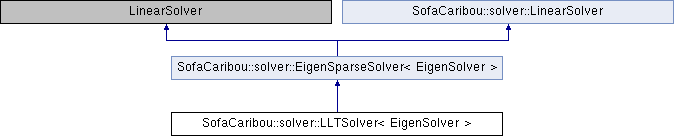Caribou
SofaCaribou::solver::LLTSolver< EigenSolver > Class Template Reference

## Detailed Description

### template<class EigenSolver> class SofaCaribou::solver::LLTSolver< EigenSolver >

Implementation of a direct LLT sparse linear solver.

This class provides a LL^T Cholesky factorizations of sparse matrices that are selfadjoint and positive definite. In order to reduce the fill-in, a symmetric permutation P is applied prior to the factorization such that the factorized matrix is P A P^-1.

The component uses the Eigen SimplicialLLT class as the solver backend.

Template Parameters
 EigenSolver Eigen direct solver type

`#include <LLTSolver.h>`

Inheritance diagram for SofaCaribou::solver::LLTSolver< EigenSolver >:## Public Types

template<typename T >
using Data = sofa::Data< T >Public Types inherited from SofaCaribou::solver::EigenSparseSolver< EigenSolver >
using EigenSolver = EigenSolver

using SparseMatrix = std::remove_cv_t< typename EigenSolver::MatrixType >

using Vector = Eigen::Matrix< FLOATING_POINT_TYPE, Eigen::Dynamic, 1 >

## Public Member Functions

SOFA_CLASS (SOFA_TEMPLATE(LLTSolver, EigenSolver), SOFA_TEMPLATE(EigenSparseSolver, EigenSolver))Public Member Functions inherited from SofaCaribou::solver::EigenSparseSolver< EigenSolver >
SOFA_CLASS (SOFA_TEMPLATE(EigenSparseSolver, EigenSolver), sofa::core::behavior::LinearSolver)

virtual void assemble (const sofa::core::MechanicalParams *mparams)
Assemble the system matrix A = (mM + bB + kK) inside the SparseMatrix p_A. More...

void resetSystem () final
Reset the complete system (A, x and b are cleared). More...

void setSystemMBKMatrix (const sofa::core::MechanicalParams *mparams) final
Set the linear system matrix A = (mM + bB + kK), storing the coefficients m, b and k of the mechanical M,B,K matrices. More...

void setSystemRHVector (sofa::core::MultiVecDerivId b_id) final
Gives the identifier of the right-hand side vector b. More...

void setSystemLHVector (sofa::core::MultiVecDerivId x_id) final
Gives the identifier of the left-hand side vector x. More...

void solveSystem () override
Solves the system using the Eigen solver.

virtual auto symmetric () const -> bool
States if the system matrix is symmetric. More...

virtual void set_symmetric (bool is_symmetric)
Explicitly states if this matrix is symmetric.

auto solver () const -> const EigenSolver &
Get a readonly reference to the backend solver.

auto solver () -> EigenSolver &
Get a reference to the backend solver.

auto mechanical_params () const -> const sofa::core::MechanicalParams &
Get a readonly reference to the mechanical parameters.

auto matrix_accessor () const -> const sofa::component::linearsolver::DefaultMultiMatrixAccessor &
Get a readonly reference to the multi-matrix accessor. More...

auto A () const -> const SparseMatrix &
Get a readonly reference to the global assembled system matrix.

auto b_id () const -> const sofa::core::MultiVecDerivId &
Get a readonly reference to the right-hand side vector identifier.

auto x_id () const -> const sofa::core::MultiVecDerivId &
Get a readonly reference to the left-hand side unknown vector identifier.

auto A_is_factorized () const -> bool
True if the solver has successfully factorize the system matrix.Public Member Functions inherited from SofaCaribou::solver::LinearSolver
virtual sofa::defaulttype::BaseMatrix * create_new_matrix (unsigned int rows, unsigned int cols) const =0
Creates a new BaseMatrix of size rows x cols. More...

virtual sofa::defaulttype::BaseVector * create_new_vector (unsigned int n) const =0
Creates a new BaseVector of size n. More...

## Static Public Member Functions

static std::string BackendName ()Static Public Member Functions inherited from SofaCaribou::solver::EigenSparseSolver< EigenSolver >
static auto GetCustomTemplateName () -> std::string

static auto canCreate (Derived *o, sofa::core::objectmodel::BaseContext *context, sofa::core::objectmodel::BaseObjectDescription *arg) -> bool

The documentation for this class was generated from the following files:
• /home/jnbrunet/sources/caribou/src/Plugin/Solver/LLTSolver.h
• /home/jnbrunet/sources/caribou/src/Plugin/Solver/LLTSolver.inl